Matplotlib Intro¶

In this reading, we'll learn how to create plots from Pandas data. Pandas uses a module called matplotlib to create plots. The matplotlib library is designed to resemble MATPLOT (a programming language for matrices and environment that support visualization).

While we could import matplotlib and make function calls directly to plot data, many Pandas methods for Series and DataFrame objects make this easier. The documentation gives a nice overview of this integration here with more examples than provided here.

Let's begin by trying to make a pie chart from a Pandas Series.

In :
import pandas as pd
from pandas import Series, DataFrame

In :
# first we'll create a Series with three numbers
s = Series([50, 30, 20])
s

Out:
0    50
1    30
2    20
dtype: int64
In :
# there are a bunch of methods of the form Series.plot.METHOD for plotting.
# we we want a pie plot:
s.plot.pie()

Out:
<matplotlib.axes._subplots.AxesSubplot at 0x119285160>

Oops! That's not what we wanted. We created a plot, but it didn't get rendered in the notebook. It turns out that matplotlib is integrated with Jupyter Notebooks, and sometimes we need a special command to tell Jupyter we want to render plots inline. Special Jupyter commands begin with a percent sign ("%"). We recommend putting the following at the beginning of all your notebooks (it's a Jupyter command, not Python code, so it won't work in a regular .py file if you were to try that):

In :
%matplotlib inline


Ok, let's try plotting again.

In :
s.plot.pie()

Out:
<matplotlib.axes._subplots.AxesSubplot at 0x11935a208>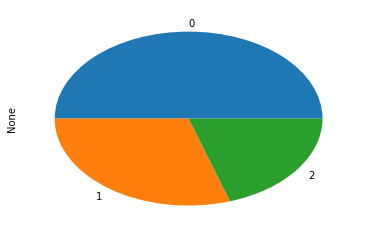Now we're getting somewhere! Of course, there are still many issues with this plot:

1. it's oval (not round)
2. each slice is numbered (not labeled)
3. it says "None" to the left

Pie Plot¶

Let's address some of the issues we just saw. First, we can pass a figsize tuple argument to specify the (width, height) in inches. Let's make the pie chart a 6-by-6 inch square.

In :
s = Series([50, 30, 20])
s

Out:
0    50
1    30
2    20
dtype: int64
In :
s.plot.pie(figsize=(6,6))

Out:
<matplotlib.axes._subplots.AxesSubplot at 0x11939ba20>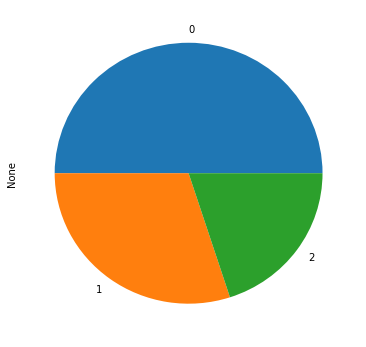Great! Now it's the right shape. What about the slice labels? These are based on the Series index. Notice that the numbers 50, 30, and 20 have indices 0, 1, and 2 respectively:

In :
s

Out:
0    50
1    30
2    20
dtype: int64

Let's give the Series custom labels and try again.

In :
s = Series([50,30,20], index=['Police', 'Schools', 'Fire'])
s.plot.pie(figsize=(6,6))

Out:
<matplotlib.axes._subplots.AxesSubplot at 0x1194384e0>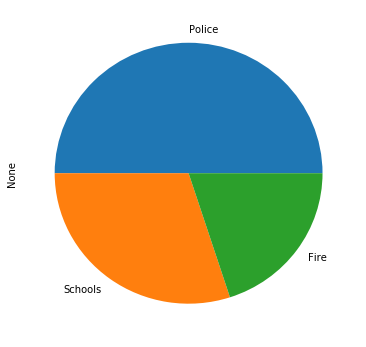The final thing to fix is the "None" label to the left. This label comes from the Series name. We haven't seen how to specify a Series name before, but it's easy:

In :
s = Series([50,30,20], index=['Police', 'Schools', 'Fire'], name='City Budget')
s

Out:
Police     50
Schools    30
Fire       20
Name: City Budget, dtype: int64

Now that the Series was given a name with name='City Budget', we will see it when we plot:

In :
s.plot.pie(figsize=(6,6))

Out:
<matplotlib.axes._subplots.AxesSubplot at 0x11952e908>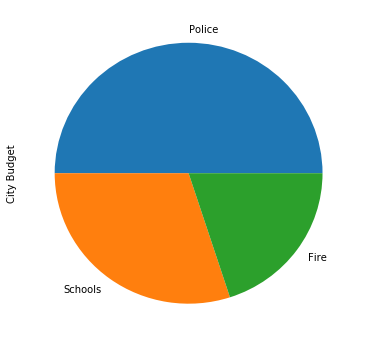We can also override using the Series name as the label by passing a label=NAME argument.

In :
s.plot.pie(figsize=(6,6), label='Percent of Budget')

Out:
<matplotlib.axes._subplots.AxesSubplot at 0x11952dcc0>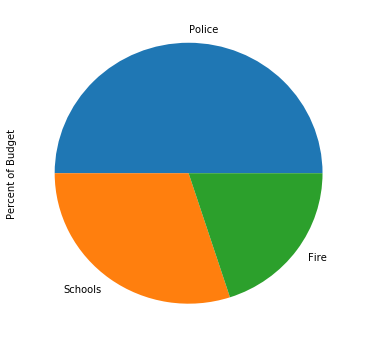This means we can also choose not to have a label:

In :
s.plot.pie(figsize=(6,6), label='')

Out:
<matplotlib.axes._subplots.AxesSubplot at 0x1196ab0f0>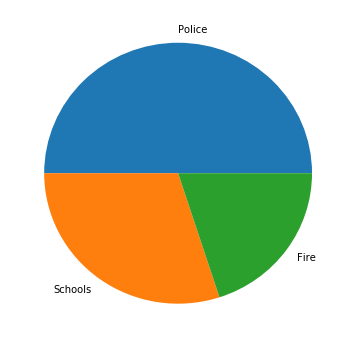Note that a label is different that a title. Let's try having a pie chart with both.

In :
s.plot.pie(figsize=(6,6), label='Percent of Budget', title='Budget Overview')

Out:
<matplotlib.axes._subplots.AxesSubplot at 0x1197675f8>One thing to be careful about is when the numbers in a Series sum to less than 1. Matplotlib does not draw a complete pie in this case.

In :
# let's say the numbers are in millions of dollars for a small town
s = Series([0.2,0.12,0.08], index=['Police', 'Schools', 'Fire'], name='City Budget')
s

Out:
Police     0.20
Schools    0.12
Fire       0.08
Name: City Budget, dtype: float64
In :
s.plot.pie(figsize=(6,6))

Out:
<matplotlib.axes._subplots.AxesSubplot at 0x119819978>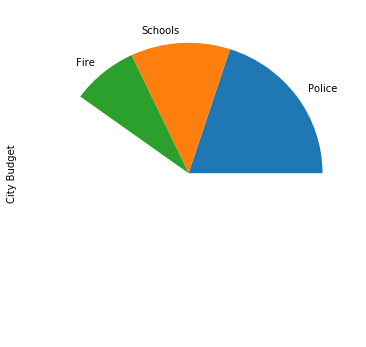This is easy enough to fix by doing some element-wise multiplication to convert everything to a percent of a total.

In :
percents = 100 * s / sum(s)
percents

Out:
Police     50.0
Schools    30.0
Fire       20.0
Name: City Budget, dtype: float64
In :
percents.plot.pie(figsize=(6,6))

Out:
<matplotlib.axes._subplots.AxesSubplot at 0x1199d6828>As a finishing touch, we may want to annotate each slice with a percentage. We can do this with the autopct=FORMAT argument. FORMAT will be a Python format string, telling matplotlib how to display the number. We haven't learned about format strings this semester, but %d will tell matplotlib to format as in integer, and %f will tell it to format as a float. Let's display integers:

In :
percents.plot.pie(figsize=(6,6), autopct='%d')

Out:
<matplotlib.axes._subplots.AxesSubplot at 0x119a19b00>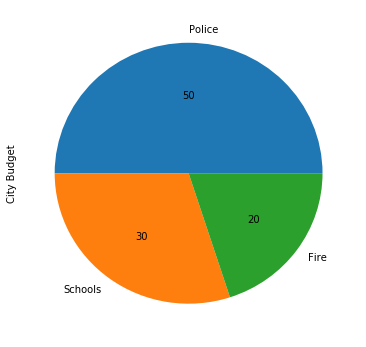In this example, we want to show how many people ride the 5 most popular bus routes in Madison, relative to overall ridership. We'll pull the data from our bus.db database we've used in previous examples.

In :
import sqlite3

In :
c = sqlite3.connect('bus.db')

In :
# let's preview the data
pd.read_sql("SELECT * from boarding LIMIT 10", c)

Out:
index StopID Route Lat Lon DailyBoardings
0 0 1163 27 43.073655 -89.385427 1.03
1 1 1163 47 43.073655 -89.385427 0.11
2 2 1163 75 43.073655 -89.385427 0.34
3 3 1164 6 43.106465 -89.340021 10.59
4 4 1167 3 43.077867 -89.369993 3.11
5 5 1167 4 43.077867 -89.369993 2.23
6 6 1167 10 43.077867 -89.369993 0.11
7 7 1167 38 43.077867 -89.369993 1.36
8 8 1169 3 43.089707 -89.329817 18.90
9 9 1169 37 43.089707 -89.329817 1.35
In :
# we want to see the total ridership per bus route
df = pd.read_sql("SELECT Route, SUM(DailyBoardings) as ridership " +
"FROM boarding " +
"GROUP BY Route " +
"ORDER BY ridership DESC", c)

# let's peek at the first few rows in the results from our query

Out:
Route ridership
0 80 10211.79
1 2 4808.03
2 6 4537.02
3 10 4425.23
4 3 2708.55

Now's a good time to stop and think about what form the data is in, and what form we want to get it to.

What we have: a DataFrame of routes and ridership, indexed from 0.

What we want: a Series of the top 5 buses, with route numbers as the index, and ridership as the values.

Why do we want such a Series? Because when we call Series.plot.pie(...) we want a pie with five slices. Each pie should be labeled as a bus route (and slice labels are pulled from the index of a Series), and the size of the series should correspond to ridership (and slice sizes are based on the values in a Series).

The first step to getting the data in the form we want is to re-index df so that the route numbers are in the index (instead of 0, 1, 2, etc). We can do this with the DataFrame.set_index function.

In :
# set_index doesn't change df, but it returns a new
# DataFrame with the desired column as the new index
ridership_df = df.set_index("Route")

Out:
ridership
Route
80 10211.79
2 4808.03
6 4537.02
10 4425.23
3 2708.55
In :
# we can pull the (only) ridership column from that DataFrame out
# and keep it as a Series.
ridership = ridership_df['ridership']

Out:
Route
80    10211.79
2      4808.03
6      4537.02
10     4425.23
3      2708.55
Name: ridership, dtype: float64

Great! Now we have the data in a plottable form. Let's make the pie chart.

In :
ridership.plot.pie()

Out:
<matplotlib.axes._subplots.AxesSubplot at 0x119f3a1d0>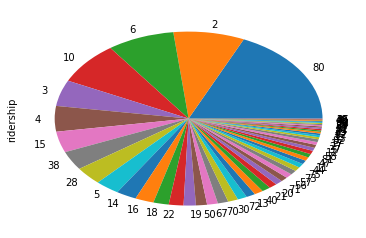This is very close to the form we want! But we only wanted the top 5 routes.

In :
ridership.head(5).plot.pie()

Out:
<matplotlib.axes._subplots.AxesSubplot at 0x119f28a58>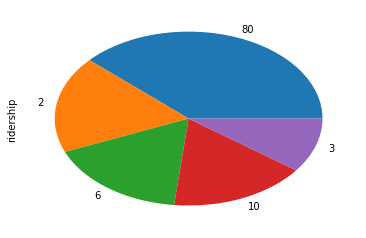Not bad, but we would ideally have an "other" category that captures all the routes besides the 80, 2, 6, 10, and 3. How many routes are in this other category?

In :
other_ridership = sum(ridership) - sum(ridership.head(5))
other_ridership

Out:
29296.560000000012

Now, we want to glue together the top five values from the ridership.head(5) series with a Series of one value (the ridership for other). We can do this with pd.concat.

In :
series1 = ridership.head()
series1

Out:
Route
80    10211.79
2      4808.03
6      4537.02
10     4425.23
3      2708.55
Name: ridership, dtype: float64
In :
series2 = Series([other_ridership], index=['other'])
series2

Out:
other    29296.56
dtype: float64
In :
plot_data = pd.concat([series1, series2])
plot_data

Out:
80       10211.79
2         4808.03
6         4537.02
10        4425.23
3         2708.55
other    29296.56
dtype: float64

That's exactly what we want! The ridership of the top 5 routes, and the remaining ridership spread across other routes. Let's plot it.

In :
plot_data.plot.pie(figsize=(6,6), autopct="%d", label="Per Route", title="Madison Metro Ridership")

Out:
<matplotlib.axes._subplots.AxesSubplot at 0x11a05efd0>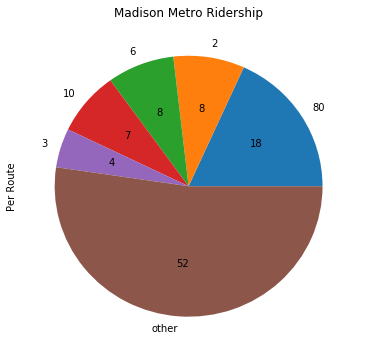This is exactly what we want. We can see the top route (the 80) is responsible for 18% of the ridership. The top 5 routes together are responsible for almost half of all ridership (48%, to be exact). To wrap up, let's make sure we close our connection to bus.db.

In :
c.close()


Scatter Plot¶

A scatter plot displays a collection of points along an x-axis and y-axis. Whereas pie charts are one-dimensional (we want to see a distribution of one value, such as ridership), scatter plots are naturally two dimensionals (each point has both an x and y position). Thus, scatter plots are generated from DataFrames (in contrast, pie charts are generated from a Series).

Just as there are a collection of Series.plot.METHOD methods, there are also a collection of DataFrame.plot.METHOD methods (scatter is one of those methods).

Let's begin by plotting some young trees. Each tree has an age (in years), a height (in feet), and a diameter (in inches).

In :
trees = [
{"age": 1, "height": 1.5, "diameter": 0.8},
{"age": 1, "height": 1.9, "diameter": 1.2},
{"age": 1, "height": 1.8, "diameter": 1.4},
{"age": 2, "height": 1.8, "diameter": 0.9},
{"age": 2, "height": 2.5, "diameter": 1.5},
{"age": 2, "height": 3, "diameter": 1.8},
{"age": 2, "height": 2.9, "diameter": 1.7},
{"age": 3, "height": 3.2, "diameter": 2.1},
{"age": 3, "height": 3, "diameter": 2},
{"age": 3, "height": 2.4, "diameter": 2.2},
{"age": 2, "height": 3.1, "diameter": 2.9},
{"age": 4, "height": 2.5, "diameter": 3.1},
{"age": 4, "height": 3.9, "diameter": 3.1},
{"age": 4, "height": 4.9, "diameter": 2.8},
{"age": 4, "height": 5.2, "diameter": 3.5},
{"age": 4, "height": 4.8, "diameter": 4},
]
df = DataFrame(trees)
df

Out:
age diameter height
0 1 0.8 1.5
1 1 1.2 1.9
2 1 1.4 1.8
3 2 0.9 1.8
4 2 1.5 2.5
5 2 1.8 3.0
6 2 1.7 2.9
7 3 2.1 3.2
8 3 2.0 3.0
9 3 2.2 2.4
10 2 2.9 3.1
11 4 3.1 2.5
12 4 3.1 3.9
13 4 2.8 4.9
14 4 3.5 5.2
15 4 4.0 4.8

Let's plot this data and see if there seems to be any connection between tree age and tree height. We can create plots like this: df.plot.scatter(x=FIELD1, y=FIELD2).

In :
# you can choose which field is represented on the x-axis
# and which is represented on the y-axis.
df.plot.scatter(x='height', y='age')

Out:
<matplotlib.axes._subplots.AxesSubplot at 0x11a16b240>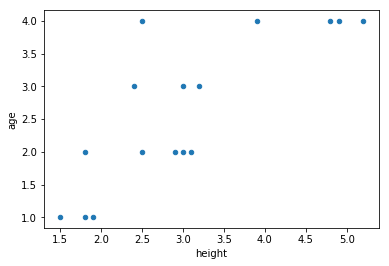Although the above plot is informative (we can see that older trees are generally taller), it's not the easiest way to visualize the information. In general, people are accustomed to seeing time-related data on the x-axis (age is a type of time). Thus, a more intuitive plot would reverse the axes:

In :
df.plot.scatter(x='age', y='height')

Out:
<matplotlib.axes._subplots.AxesSubplot at 0x11a254dd8>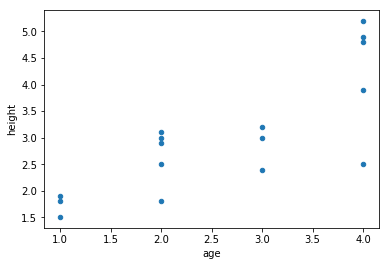We can also control the color (with the c argument) and size (with the s argument) of the points:

In :
df.plot.scatter(x='age', y='height', c='black', s=50)

Out:
<matplotlib.axes._subplots.AxesSubplot at 0x11a0256a0>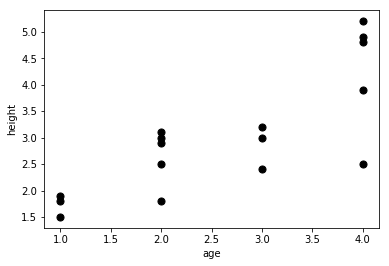Visually Communicating more Attributes¶

If we want, we can also use our data to determine the size and color of each point by passing a series for these. For example, suppose we want tall trees to be represented by large, black circles, and we wanted short trees to be represted with small gray dots. We can pull out a display Series to control this.

In :
display = df['height'] * 25
display

Out:
0      37.5
1      47.5
2      45.0
3      45.0
4      62.5
5      75.0
6      72.5
7      80.0
8      75.0
9      60.0
10     77.5
11     62.5
12     97.5
13    122.5
14    130.0
15    120.0
Name: height, dtype: float64
In :
df.plot.scatter(x='age', y='height', c=display, s=display)

Out:
<matplotlib.axes._subplots.AxesSubplot at 0x11a317a20>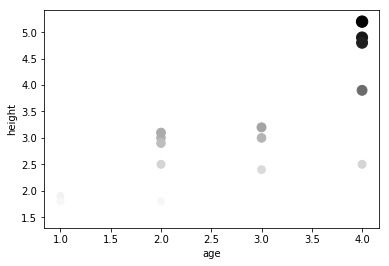Bigger numbers in the display Series (or any Series used with c=) results in darker dots, and bigger numbers also determine the size of the dots.

The above plot is an example of a reduntant visualization. It's reduntant because three characteristics of the plot (x-axis, dot color, and dot size) are all being used to communicate the same characteristic of the data (height). This is a wasteful use of plot characteristics, considering that we didn't communicate anything about tree diameter.

When you're thinking about how to visualize your data, you should list the interesting attributes of your data as well as the dimensions you can communicate with the plot. Then carefully consider how to use each dimension of your plot to communicate something interesting.

In this case, we have three attributes in our data:

1. tree age
2. tree height
3. tree diameter

We also have four dimensions we can control in our plot:

1. x-axis
2. y-axis
3. dot size
4. dot color

Which dimensions should communicate which attributes? Exact opinions will vary, but some combinations are more effective than others.

One good combination will be x-axis for age, y-axis for height, and dot size for diameter. As mentioned before, the x-axis is often used to communicate an elapsed time (an age). It also feels very natural for a vertical axis to communicate height. Finally, dot size seems like a better fit for diameter than color for two reasons. First, it is intuitive to pair a spatial attribute with a spatial characteristic of the plot. Second, color is often a tricky dimension to use. Gray can be too light to see, printed copies will very in how good they look, and finally you need to think about how accessible your plots will be for color-blind readers.

Let's see how the data looks with our final choices:

In :
df.plot.scatter(x='age', y='height', s=df['diameter'], c='black')

Out:
<matplotlib.axes._subplots.AxesSubplot at 0x11a4af128>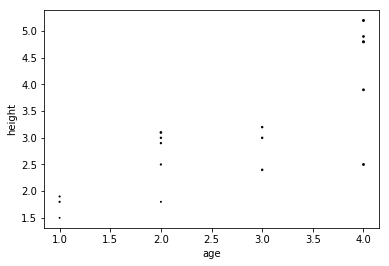Those dots are a little small, and the visualization is relative (there's no scale specifying what a given dot size means), so we're free to multiply the diameter by a number of our choosing to make it more aesthetically pleasing.

In :
df.plot.scatter(x='age', y='height', s=df['diameter'] * 40, c='black')

Out:
<matplotlib.axes._subplots.AxesSubplot at 0x11a4a3908>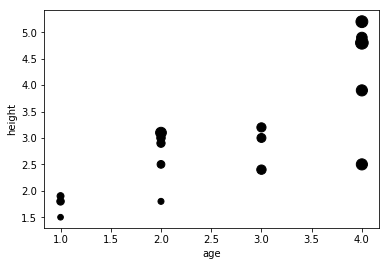Example: Iris Data¶

An Iris is a type of flowering plant. There is a very popular dataset (often used in machine learning examples) containing a description of the dimensions of 150 Iris plants from 3 different types of Iris.

The dataset is at https://archive.ics.uci.edu/ml/machine-learning-databases/iris/iris.data, and a description of the data is here. We will plot the data for each of the three types of Iris to visually identify patterns in the data.

As a first step, let's try fetching and loading the CSV data.

In :
df = pd.read_csv('https://archive.ics.uci.edu/ml/machine-learning-databases/iris/iris.data')

Out:
5.1 3.5 1.4 0.2 Iris-setosa
0 4.9 3.0 1.4 0.2 Iris-setosa
1 4.7 3.2 1.3 0.2 Iris-setosa
2 4.6 3.1 1.5 0.2 Iris-setosa
3 5.0 3.6 1.4 0.2 Iris-setosa
4 5.4 3.9 1.7 0.4 Iris-setosa

Oops! There's no CSV header in that file, so we have to tell Pandas what each field means. From the documentation, we see the following:

1. sepal length in cm
2. sepal width in cm
3. petal length in cm
4. petal width in cm
5. class:
-- Iris Setosa
-- Iris Versicolour
-- Iris Virginica

Let's try again using header=.

In :
df = pd.read_csv('https://archive.ics.uci.edu/ml/machine-learning-databases/iris/iris.data',
names=['sepal-len', 'sepal-wid', 'petal-len', 'petal-wid', 'name'])

Out:
sepal-len sepal-wid petal-len petal-wid name
0 5.1 3.5 1.4 0.2 Iris-setosa
1 4.9 3.0 1.4 0.2 Iris-setosa
2 4.7 3.2 1.3 0.2 Iris-setosa
3 4.6 3.1 1.5 0.2 Iris-setosa
4 5.0 3.6 1.4 0.2 Iris-setosa

Great! Now lets see how many flowers there are of each type.

In :
iris_types = ['Iris-setosa', 'Iris-versicolor', 'Iris-virginica']

for name in iris_types:
rows = df[df['name'] == name]
print(name, len(rows))

Iris-setosa 50
Iris-versicolor 50
Iris-virginica 50

In :
for name in iris_types:
rows = df[df['name'] == name]
rows.plot.scatter(x='petal-len', y='petal-wid', title=name)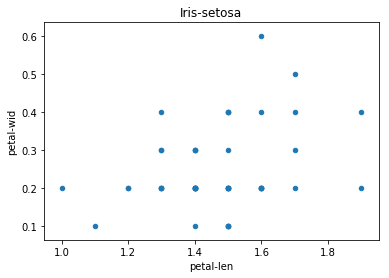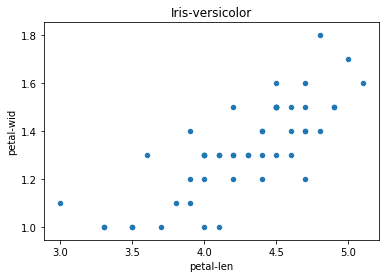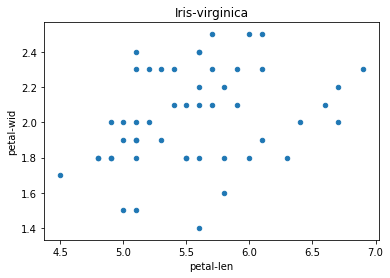What if we want just one plot showing all three flower types? In order to distinguish, we would want the dots for each flower type to be a different color.

It turns out that whenever we call a DataFrame.plot.METHOD method, it returns an AxesSubplot object. An AxesSubplot object represents the area where we're displaying our data. If we pass it to other DataFrame.plot.METHOD methods, we can indicate that we want the newly plotted data to appear in the same region where we previously plotted. The argument for this is called ax, short for AxesSubplot. Let's try it.

In :
ax = df[df['name'] == 'Iris-setosa'].plot.scatter(x='petal-len', y='petal-wid', c='blue')
df[df['name'] == 'Iris-versicolor'].plot.scatter(x='petal-len', y='petal-wid', c='green', ax=ax)
df[df['name'] == 'Iris-virginica'].plot.scatter(x='petal-len', y='petal-wid', c='black', ax=ax)

Out:
<matplotlib.axes._subplots.AxesSubplot at 0x11a7c1f60>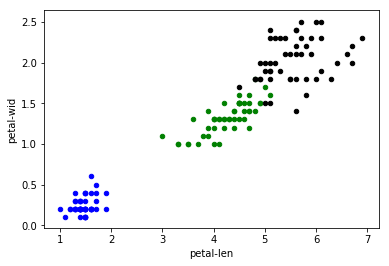From this plot, we can make several observations:

1. petal length is generally correlated with petal width
2. the Setosa variety is smallest along both dimensions
3. the Virginica variety is largest along both dimensions

What about sepal size? We leave that as an exercise for you!

Conclusion¶

In this reading, we have learned how to create a pie chart from a Pandas Series and a scatter plot from a Pandas DataFrame. We have also discussed the decision making process for choosing which plot characteristics should represent which data attributes. Finally, we learned how about AxesSuplot objects and how to use them to plot multiple datasets in the same region. We did all of this in the context of two example datasets: the Madison Metro dataset and the popular Iris dataset.